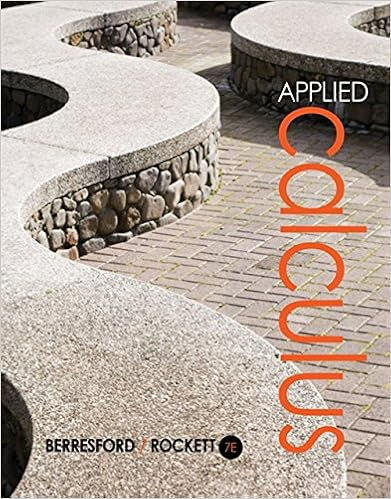# Youre favorite mms are red so you want to create a

• Homework Help
• 64

This preview shows page 18 - 23 out of 64 pages.

##### We have textbook solutions for you!
The document you are viewing contains questions related to this textbook.The document you are viewing contains questions related to this textbook.
Chapter 11 / Exercise 7
Applied Calculus
Berresford/RockettExpert Verified
5.You’re favorite M&M’s are red, so you want to create a simulation for modeling the drawing of red M&M’s from a bag with the color probabilities as listed above. Describe a simulation. Remember: you are only trying to simulate drawing a red M&M.
##### We have textbook solutions for you!
The document you are viewing contains questions related to this textbook.The document you are viewing contains questions related to this textbook.
Chapter 11 / Exercise 7
Applied Calculus
Berresford/RockettExpert Verified
42 7.1d Class Activity: More Models and Probability Win the Spin!Determine the probability that Player 1 wins the spin (highest number wins). Player 1 uses spinner A and Player 2 uses spinner B. Assume that the areas on each spinner are equal in size. 1.Create a probability model for the outcomes of the “Win the Spin” game, using a tree diagram. Spinner A438591Outcomes Probability 2.How many possible outcomes are there? How do you know? 3.What is the probability of each of the outcomes? How do you know? 4.What is the probability that Player 1 will win? How do you know? 5.What is the probability that Player 2 will win? How do you know? 6.What is the probability of a draw (tie)? How do you know? 7.Is this a fair game? Why or why not? 8.If you were to play the game, your outcome would not necessarily match the probabilities above. Explain why this is true. Spinner BSpinner A438591
9.Create a probability model for the outcomes of the “Odd or Even” game, using a tree diagram. 10.Use the tree diagram to figure out if the game is fair or not. Explain. 11.Use the rule for calculating compound probability to calculate the probabilities of the different combinations of the spins for Players 1 and 2. Show all your work.
44 redgreenbluered7.1d Homework: Probability Models and Spinner Games 1.The spinner at right is spun twice. List the sample space for the possible outcomes from two spins. Use set notation. (Hint: there are 9 outcomes!) S = 2.Are all the outcomes in the sample space equally likely? Why or why not? 3.How might you figure out the number of outcomes without making a list or diagram? Explain. 4.Create a probability model for the outcomes for spinning the spinner twice (organized list, tree diagram, or table) to show all possible outcomes and probabilities from two spins of the spinner. 5.Fill in the spaces below and make a conjecture about a rule for the number of possible outcomes for compound events. If there are 3 possible outcomes in the first event, and 2 possible outcomes in the second event, then there will be _______ possible outcomes in the compound event. If there are 5 possible outcomes in the first event, and 3 possible outcomes in the second event, then there will be _______ possible outcomes in the compound event. If there are “a”possible outcomes in the first event, and “b”possible outcomes in the second event, then there will be ________ possible outcomes in the compound event.
45 6.Examine the model you created above to determine these probabilities.
•••